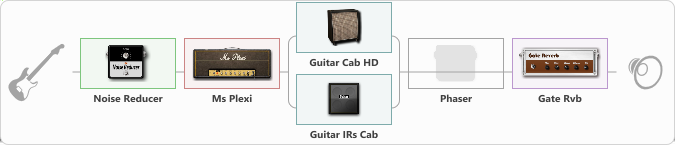# Early 70s

Discussion in 'ToneLib-GFX presets' started by Dominique Sideleau, Nov 5, 2022.

1. Early 70s

Preset name: Early 70s

Effects chain:Effect: "Noise Reducer" (Dynamics / Filter), active - "yes"
{
"Sens" = 28
"Mode" = Soft
}

Effect: "Ms Plexi" (Amp simulators), active - "yes"
{
"Gain" = 24
"Bass" = 50
"Middle" = 46
"Treble" = 51
"Presence" = 20
"Master" = 74
"Level (dB)" = 5
}

Effect: "Splitter" (Dynamics / Filter)
{
"A-Bypass" = Off
"A-Pan" = -25
"A-Level" = 55
"B-Bypass" = Off
"B-Pan" = 25
"B-Level" = 55
"Width" = 0

'A' branch:
{

Effect: "Guitar Cab HD" (Cabinets), active - "yes"
{
"Model" = Marshall 1960A (4x12")
"Mic Model" = Dynamic SM57
"Mic Position" = Center
"Mic Distance" = Middle
"Low Cut (Hz)" = 57
"Hi Cut (kHz)" = 15.5
"Mix" = 54
"Level (dB)" = 0
}
}
'B' branch:
{

Effect: "Guitar IRs Cab" (Cabinets), active - "yes"
{
"Model" = Marshall 1960b (4x12")
"Mic Position" = Middle
"Mic Distance" = Middle
"Low Cut (Hz)" = 205
"Hi Cut (kHz)" = 16.0
"Mix" = 49
"Level (dB)" = 0
}
}
}

Effect: "Phaser" (Modulation / Sfx), active - "no"
{
"Model" = MXR Phase 90
"Speed" = 2.7
"Depth" = 39
"Reso" = 24
"Freq" = 2.6
}

Effect: "Gate Rvb" (Reverberation), active - "yes"
{
"Time" = 111
"PreDelay" = 0
"LoDamp" = 15
"HiDamp" = 82
"Type" = Convent
"Mix" = 29
}

Note: You will need to download and install the ToneLib-GFX software to use the preset.

File size:
737 bytes
Views:
2,755